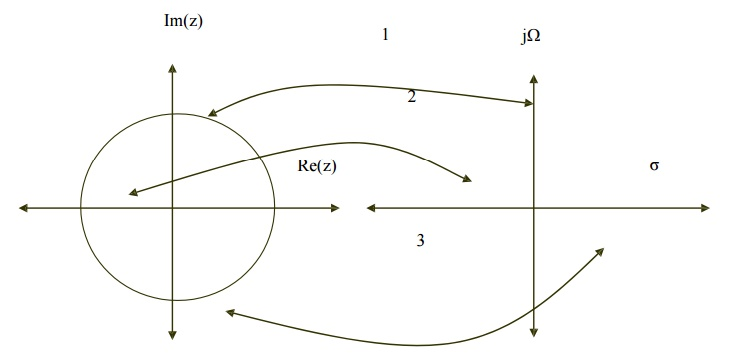Home | | Discrete Time Systems and Signal Processing | Structures For IIR Systems

# Structures For IIR Systems

IIR Systems are represented in four different ways 1. Direct Form Structures Form I and Form II 2. Cascade Form Structure 3. Parallel Form Structure 4. Lattice and Lattice-Ladder structure.

STRUCTURES FOR IIR SYSTEMS

IIR Systems are represented in four different ways

1. Direct Form Structures Form I and Form II

3. Parallel Form Structure

1.

DIRECT FORM STRUCTURE FOR IIR SYSTEMS

IIR systems can be described by a generalized equations asOverall IIR system can be realized as cascade of two function H1(z) and H2(z). Here H1(z) represents zeros of H(z) and H2(z) represents all poles of H(z).FIG - DIRECT FORM I REALIZATION OF IIR SYSTEM

1.     Direct form I realization of H(z) can be obtained by cascading the realization of H1(z) which is all zero system first and then H2(z) which is all pole system.

2.     There are M+N-1 unit delay blocks. One unit delay block requires one memory location. Hence direct form structure requires M+N-1 memory locations.

3.     Direct Form I realization requires M+N+1 number of multiplications and M+N number of additions and M+N+1 number of memory locations.

DIRECT FORM - II

1. Direct form realization of H(z) can be obtained by cascading the realization of H1(z) which is all pole system and H2(z) which is all zero system.

2. Two delay elements of all pole and all zero system can be merged into single delay element.

3. Direct Form II structure has reduced memory requirement compared to Direct form I structure. Hence it is called canonic form.

4. The direct form II requires same number of multiplications(M+N+1) and additions (M+N) as that of direct form I.CASCADE FORM STRUCTURE FOR IIR SYSTEMS

In cascade form, stages are cascaded (connected) in series. The output of one system is input to another. Thus total K number of stages are cascaded. The total system function 'H' is given by

H= H1(z) . H2(z)……………………. Hk(z)                   (1)

H= Y1(z)/X1(z). Y2(z)/X2(z). ……………Yk(z)/Xk(z)         (2)Each H1(z), H2(z)… etc is a second order section and it is realized by the direct form as shown in below figure.

System function for IIR systemsThus Direct form of second order IIR system is shown asPARALLEL FORM STRUCTURE FOR IIR SYSTEMS

System function for IIR systems is given asThe above system function can be expanded in partial fraction as follows

H(z) = C + H1(z) + H2(z)…………………….+ Hk(z)                     (3)

Where C is constant and Hk(z) is given asIIR FILTER DESIGN

IMPULSE INVARIANCE

BILINEAR TRANSFORMATION

BUTTERWORTH APPROXIMATION

IIR FILTER DESIGN - IMPULSE INVARIANCE METHOD

Impulse Invariance Method is simplest method used for designing IIR Filters. Important Features of this Method are

1. In impulse variance method, Analog filters are converted into digital filter just by replacing unit sample response of the digital filter by the sampled version of impulse response of analog filter. Sampled signal is obtained by putting t=nT hence

h(n) = ha(nT)                                                       n=0,1,2. ………….

where h(n) is the unit sample response of digital filter and T is sampling interval.

2. But the main disadvantage of this method is that it does not correspond to simple algebraic mapping of S plane to the Z plane. Thus the mapping from analog frequency to digital frequency is many to one. The segments

(2k-1)∏/T ≤ Ω ≤ (2k+1) ∏/T of j Ω axis are all mapped on the unit circle ∏≤ω≤∏. This takes place because of sampling.

3. Frequency aliasing is second disadvantage in this method. Because of frequency aliasing, the frequency response of the resulting digital filter will not be identical to the original analog frequency response.

4. Because of these factors, its application is limited to design low frequency filters like LPF or a limited class of band pass filters.

RELATIONSHIP BETWEEN Z PLANE AND S PLANE

Z is represented as re in polar form and relationship between Z plane and S plane is given as Z=eST where s= σ + j Ω.Here we have three condition

1.     If σ = 0 then r=1

2.     If σ < 0 then 0 < r < 1

3.     If σ > 0 then r> 1

Thus

1.     Left side of s-plane is mapped inside the unit circle.

2.     Right side of s-plane is mapped outside the unit circle.

3.     jΩ axis is in s-plane is mapped on the unit circle.Study Material, Lecturing Notes, Assignment, Reference, Wiki description explanation, brief detail
Digital Signal Processing : IIR Filter Design : Structures For IIR Systems |